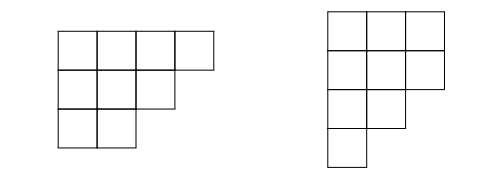# Tableaux

##### Definition: Young Diagram

A Young diagram of size n is a sequence$\lambda_1 \geq \lambda_2 \geq \cdots$ of non- negative integers such that$\sum_i \lambda_i = n$. We denote this by$\lambda$ and draw it as a collection of unit boxes where each$\lambda_i$ is the length of a row.

The length$l(\lambda)=sup\{k \geq 1 | \lambda_k >0\}$ is the number of rows in$\lambda$. We denote the set of all Young diagrams by$\mathbb{Y}$, including the empty diagram$\emptyset=(0,0, \ldots)$.

Below we see the Young diagram [4,3,2] on the left with its transpose on the right.##### Definition: Young Tableau

A semi-standard Young tableau of shape$\lambda$ and rank N is a filling of boxes of a Young diagram$\lambda$ with numbers from 1 to N such that the numbers strictly increase along the columns and weakly increase along the rows.

##### Definition: Domino Diagram

A domino diagram is a Young diagram that can be tiled by combinations of 2x1 and 1x2 rectangles (which we call dominoes)

##### Definition: Domino Tableau

A domino tableau with shape$\lambda$ and rank$N$ is a filling of dominoes of a tiling of a domino diagram$\lambda$ with numbers from 1 to N such that the numbers strictly increase along the columns and weakly increase along the rows.

##### Definition: Schur Polynomial

For a given Young Diagram$\lambda$, we defined the corresponding Schur polynomial by$$s_{\lambda}(X) = \sum_{P: sh(P)=\lambda} {\textbf{X}}^{weight(P)},$$

If the content of P has$c_1$ 1s,$c_2$ 2s, ... ,$c_n$ ns, then${\textbf{x}}^{weight(P)} := \Pi_{i=1}^n x_i^{c_i}$.